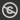Science, Maths & Technology
Free course

# Introduction to linear equations and matrices## Course reviews

In this free course, matrices are used as a concise way of representing systems of linear equations which occur frequently in mathematics. Section 1 looks at simultaneous linear equations in two and three unknowns and then generalises the ideas to systems of linear equations. Section 2 develops a strategy for solving systems of linear equations. Section 3 looks at the algebra of matrices and shows that matrices can be thought of as a generalisation of vectors. Section 4 introduces the inverse of a matrix. Section 5 uses systems of linear equations to introduce the determinant of a square matrix.

## Course learning outcomes

After studying this course, you should be able to:

• use the method of Gauss-Jordan elimination to find the solutions of systems of simultaneous linear equations
• solve a system of linear equations by row-reducing its augmented form
• perform the matrix operations of addition, multiplication and transposition and express a system of simultaneous linear equations in matrix form
• determine whether or not a given matrix is invertible and if it is, find its inverse
• evaluate the determinant of a 2 x 2 or 3 x 3 matrix and use the determinant to determine whether or not a given 2 x 2 matrix is invertible.

First Published: 08/12/2017

Updated: 18/04/2018

Skip Rate and Review